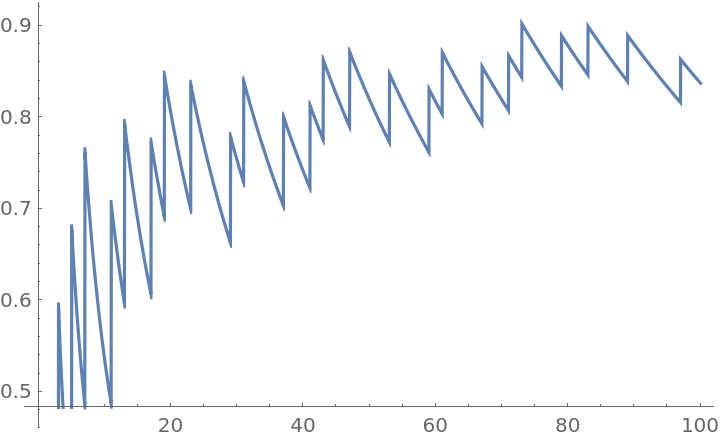Function Repository Resource:

ChebyshevTheta

Calculate the value of the first Chebyshev function θ(x)

Contributed by: Jack Heimrath
 ResourceFunction["ChebyshevTheta"][x] returns the value of the first Chebyshev function at x.

Details and Options

The first Chebyshev function θ(x) is equal to the sum of the logarithms of prime numbers less than or equal to x. For x<2 the sum is empty and by convention we set θ(x)=0.
The Prime Number Theorem is equivalent to the statement that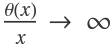as x.
θ(x)=ψ(x)+O(1), where ψ is the second Chebyshev function.

Examples

Basic Examples

Compute θ(30):

 In:=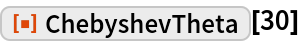Out=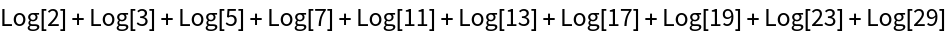Obtain a decimal approximation:

 In:=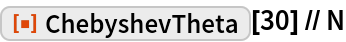Out=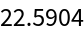Plot θ(x) from 0 to 30:

 In:=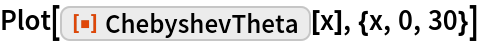Out=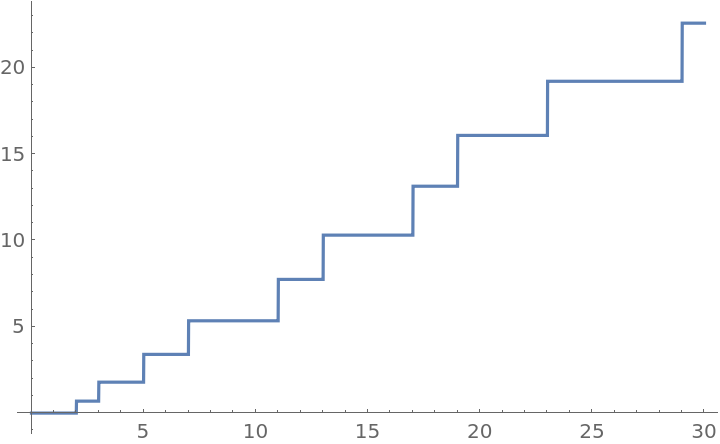Compare x, θ(x), and |x-θ(x)|:

 In:=Out=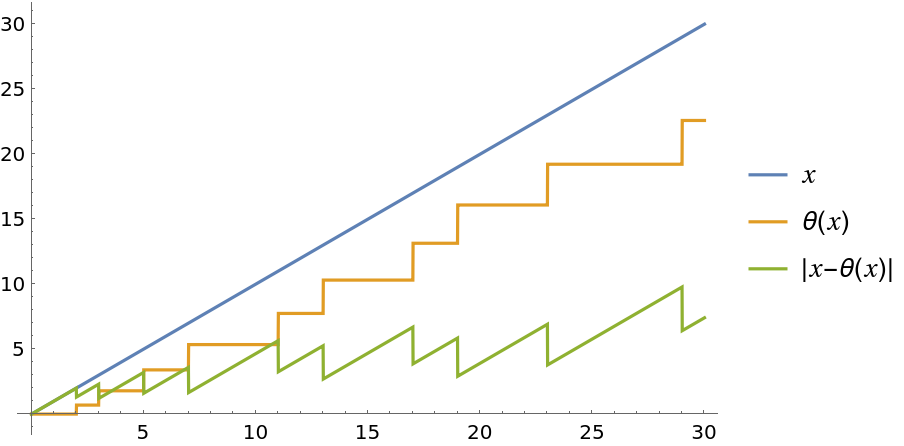Plot the ratio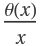:

 In:=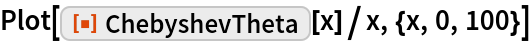Out=